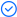WPS Office

Free All-in-One Office Suite with PDF EditorRead, edit, and convert PDFs with the powerful PDF toolkit.Microsoft-like interface, easy to use.

Windows • MacOS • Linux • iOS • Android# Display the unit symbol in the formula result

Uploaded time: January 24, 2022 Difficulty Beginner

# Display the unit symbol in the formula resultDisplay the unit symbol in the formula result

This is a table of information about the department employees. If we need to calculate the average weight of the staff, what should we do?

Normally, you only need to enter the formula =AVERAGE(D2:D8) in cell D9 to get the average.

However, the formula returns 0, and obviously, the result is incorrectbecause there are text characters in the cells, which affects the accuracy of the calculation.

Do you know how to retain the weight unit and normally perform the calculation at the same time?

At this time, we can customize the cell format to solve this problem.

Select the range of cells to which we want to apply the calculation, and press the shortcut Ctrl+1. In the pop-up dialog, select Custom.

Enter the formula 0kg in the edit box, where 0 represents the numeric placeholder, and double quotes represent the quoted text.

Then click to confirm.  When we enter the input number, the unit symbol kg will be added after the number automatically .

Enter the formula =AVERAGE(D2:D8) in cell D9, and now you can get the correct calculation result with the weight unit.

What a practical feature! Did you get it?